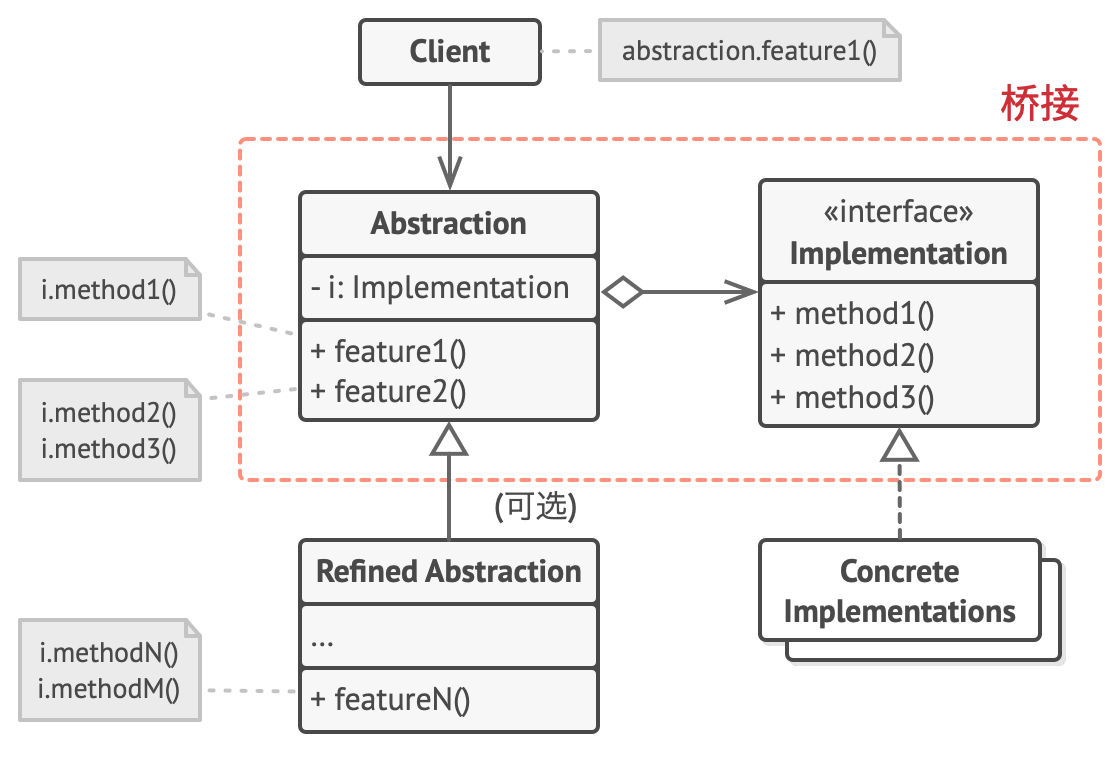# 桥接模式``````class RemoteControl {
protected device: Device;

// 遥控器基类声明了一个指向设备对象的引用成员变量。
// 所有遥控器通过通用设备接口与设备进行交互，使得同一个遥控器可以支持不同类型的设备。
constructor(device: Device) {
this.device = device;
}

togglePower(): void {
this.device.isEnabled() ? this.device.disable() : this.device.enable();
}

volumeDown(): void {
this.device.setVolume(this.device.getVolume() - 10);
}

volumeUp(): void {
this.device.setVolume(this.device.getVolume() + 10);
}

channelDown(): void {
this.device.setChannel(this.device.getChannel() - 1);
}

channelUp(): void {
this.device.setChannel(this.device.getChannel() + 1);
}
}
``````

``````interface Device {
isEnabled: () => boolean;
disable: () => void;
enable: () => void;
setVolume: (percent: number) => void;
getVolume: () => number;
setChannel: (channel: number) => void;
getChannel: () => number;
}
``````

``````class Tv implements Device {
// ...
}

class Radio implements Device {
// ...
}
``````

``````const tv = new Tv()
const remote = new RemoteControl(tv)
remote.togglePower()
``````

``````class AdvancedRemoteControl extends RemoteControl {
mute() {
this.device.setVolume(0);
}
}
``````

## 练习

``````/** 实现部分接口 */
interface Color {
drawAPI: (width: number, height: number, radius?: number) => void
}

/** 抽象部分基类 */
class Shape {
protected color: Color

// 添加指向颜色对象的引用
constructor(color: Color) {
this.color = color
}

draw() {}
}

/** 遵循实现部分接口来具体实现颜色子类 */
class Red implements Color {
drawAPI(width: number, height: number, radius?: number) {
console.log(`color: red, width: \${width}, height: \${height}, radius: \${radius}`)
}
}

class Circle extends Shape {
private width: number
private height: number

constructor(width: number, height: number, radius: number, color: Color) {
super(color)
this.width = width
this.height = height
}

draw() {
}
}

class Square extends Shape {
private width: number
private height: number

constructor(width: number, height: number, color: Color) {
super(color)
this.width = width
this.height = height
}

draw() {
this.color.drawAPI(this.width, this.height, 0)
}
}

const redCircel = new Circle(10, 10, 10, new Red())
redCircel.draw()

const redSquare = new Square(5, 5, new Red())
redSquare.draw()

class Blue implements Color {
drawAPI(width: number, height: number, radius?: number) {
console.log(`color: blue, width: \${width}, height: \${height}, radius: \${radius}`)
}
}

const blueCircle = new Circle(5, 5, 5, new Blue())
blueCircle.draw()

const blueSquare = new Square(5, 5, new Blue())
blueSquare.draw()
``````